Stacking in Machine Learning

• Last Updated : 21 Dec, 2021

Stacking:
Stacking is a way of ensembling classification or regression models it consists of two-layer estimators. The first layer consists of all the baseline models that are used to predict the outputs on the test datasets. The second layer consists of Meta-Classifier or Regressor which takes all the predictions of baseline models as an input and generate new predictions.
Stacking Architecture: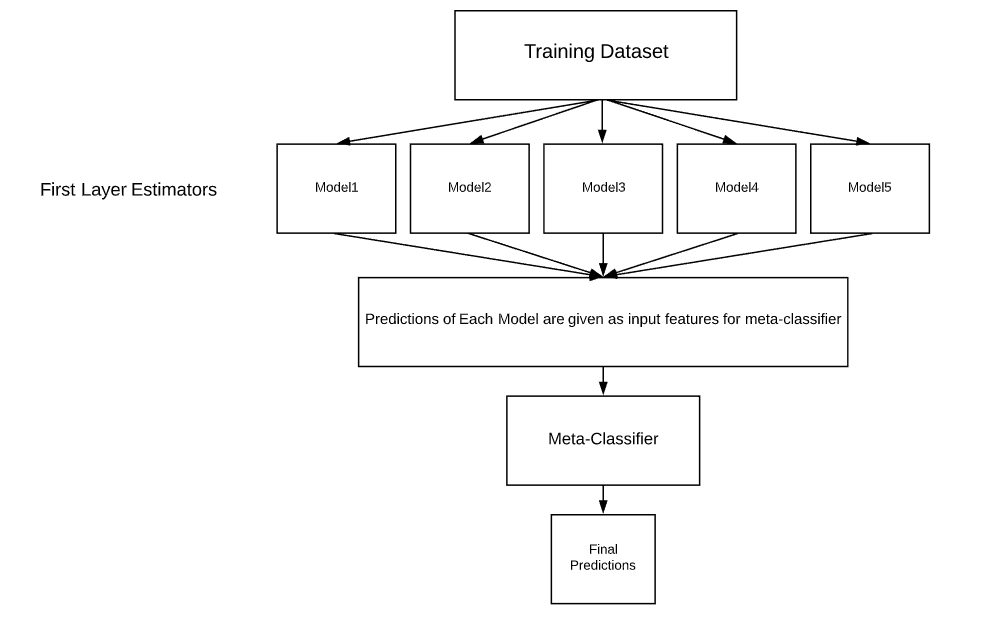Stacking Architecture

mlxtend:
Mlxtend (machine learning extensions) is a Python library of useful tools for day-to-day data science tasks. It consists of lots of tools that are useful for data science and machine learning tasks for example:

1. Feature Selection
2. Feature Extraction
3. Visualization
4. Ensembling

and many more.
This article explains how to implement Stacking Classifier on the classification dataset.
Why Stacking?
Most of the Machine-Learning and Data science competitions are won by using Stacked models. They can improve the existing accuracy that is shown by individual models. We can get most of the Stacked models by choosing diverse algorithms in the first layer of architecture as different algorithms capture different trends in training data by combining both of the models can give better and accurate results.
Installation of libraries on the system:

pip install mlxtend
pip install pandas
pip install -U scikit-learn

Code: Import Required Libraries:

python3

 import pandas as pdimport matplotlib.pyplot as pltfrom mlxtend.plotting import plot_confusion_matrixfrom mlxtend.classifier import StackingClassifierfrom sklearn.model_selection import train_test_splitfrom sklearn.preprocessing import StandardScalerfrom sklearn.linear_model import LogisticRegressionfrom sklearn.neighbors import KNeighborsClassifierfrom sklearn.naive_bayes import GaussianNBfrom sklearn.metrics import confusion_matrixfrom sklearn.metrics import accuracy_score

python3

Output: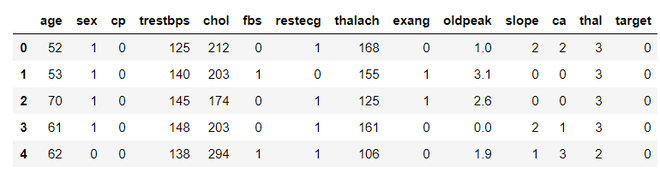Code:

python3

 # Creating X and y for trainingX = df.drop('target', axis = 1)y = df['target']

Code: Splitting Data into Train and Test

python3

 # 20 % training dataset is considered for testingX_train, X_test, y_train, y_test = train_test_split(X, y, test_size = 0.2, random_state = 42)

Code: Standardizing Data

python3

 # initializing sc objectsc = StandardScaler() # variables that needed to be transformedvar_transform = ['thalach', 'age', 'trestbps', 'oldpeak', 'chol']X_train[var_transform] = sc.fit_transform(X_train[var_transform])   # standardizing training dataX_test[var_transform] = sc.transform(X_test[var_transform])            # standardizing test dataprint(X_train.head())

Output: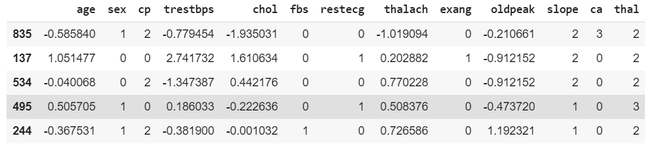Code: Building First Layer Estimators

python3

 KNC = KNeighborsClassifier()   # initialising KNeighbors ClassifierNB = GaussianNB()              # initialising Naive Bayes

Let’s Train and evaluate with our first layer estimators to observe the difference in the performance of the stacked model and general model
Code: Training KNeighborsClassifier

python3

 model_kNeighborsClassifier = KNC.fit(X_train, y_train)   # fitting Training Setpred_knc = model_kNeighborsClassifier.predict(X_test)   # Predicting on test dataset

Code: Evaluation of KNeighborsClassifier

python3

 acc_knc = accuracy_score(y_test, pred_knc)  # evaluating accuracy scoreprint('accuracy score of KNeighbors Classifier is:', acc_knc * 100)

Output: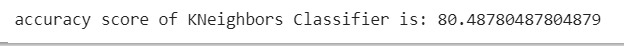Code: Training Naive Bayes Classifier

python3

 model_NaiveBayes = NB.fit(X_train, y_train)pred_nb = model_NaiveBayes.predict(X_test)

Code: Evaluation of Naive Bayes Classifier

python3

 acc_nb = accuracy_score(y_test, pred_nb)print('Accuracy of Naive Bayes Classifier:', acc_nb * 100)

Output: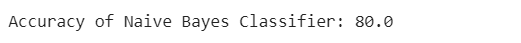Code: Implementing Stacking Classifier

python3

 lr = LogisticRegression()  # defining meta-classifierclf_stack = StackingClassifier(classifiers =[KNC, NB], meta_classifier = lr, use_probas = True, use_features_in_secondary = True)
• use_probas=True indicates the Stacking Classifier uses the prediction probabilities as an input instead of using predictions classes.
• use_features_in_secondary=True indicates Stacking Classifier not only take predictions as an input but also uses features in the dataset to predict on new data.

Code: Training Stacking Classifier

python3

 model_stack = clf_stack.fit(X_train, y_train)   # training of stacked modelpred_stack = model_stack.predict(X_test)       # predictions on test data using stacked model

Code: Evaluating Stacking Classifier

python3

 acc_stack = accuracy_score(y_test, pred_stack)  # evaluating accuracyprint('accuracy score of Stacked model:', acc_stack * 100)

Output: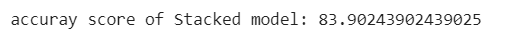Our both individual models scores an accuracy of nearly 80% and our Stacked model got an accuracy of nearly 84%.By Combining two individual models we got a significant performance improvement.
Code:

python3

 model_stack = clf_stack.fit(X_train, y_train)   # training of stacked modelpred_stack = model_stack.predict(X_test)       # predictions on test data using stacked model

Code: Evaluating Stacking Classifier

python3

 acc_stack = accuracy_score(y_test, pred_stack)  # evaluating accuracyprint('accuracy score of Stacked model:', acc_stack * 100)

Output:Our both individual models scores an accuracy of nearly 80% and our Stacked model got an accuracy of nearly 84%. By Combining two individual models we got a significant performance improvement.

My Personal Notes arrow_drop_up